## Help me please!! An emergency!! A polynomial equation with rational coefficients has the roots 5, sqrt6, 3, -sqrt7. Find two ad

Question

A polynomial equation with rational coefficients has the roots 5, sqrt6, 3, -sqrt7. Find two additional roots.

Thank you!

in progress 0
2 weeks 2021-08-28T18:22:53+00:00 1 Answers 0 views 0

1. Given:

A polynomial equation with rational coefficients has the roots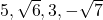.

To find:

According to the irrational root theorem, if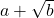is a root of a polynomial, then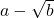is also the root of that polynomial.
It is given that,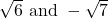are roots of a polynomial.
By using irrational root theorem,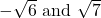are also the roots of that polynomial.
Therefore, the two additional roots are.